# 第 3 章 Python 的数据结构、函数和文件

## 3.1 数据结构和序列

Python 的数据结构简单而强大。通晓它们才能成为熟练的 Python 程序员。

### 元组

In : tup = 4, 5, 6

In : tup
Out: (4, 5, 6)


In : nested_tup = (4, 5, 6), (7, 8)

In : nested_tup
Out: ((4, 5, 6), (7, 8))


tuple可以将任意序列或迭代器转换成元组：

In : tuple([4, 0, 2])
Out: (4, 0, 2)

In : tup = tuple('string')

In : tup
Out: ('s', 't', 'r', 'i', 'n', 'g')


In : tup
Out: 's'


In : tup = tuple(['foo', [1, 2], True])

In : tup = False
---------------------------------------------------------------------------
TypeError                                 Traceback (most recent call last)
<ipython-input-10-c7308343b841> in <module>()
----> 1 tup = False
TypeError: 'tuple' object does not support item assignment


In : tup.append(3)

In : tup
Out: ('foo', [1, 2, 3], True)


In : (4, None, 'foo') + (6, 0) + ('bar',)
Out: (4, None, 'foo', 6, 0, 'bar')


In : ('foo', 'bar') * 4
Out: ('foo', 'bar', 'foo', 'bar', 'foo', 'bar', 'foo', 'bar')


### 拆分元组

In : tup = (4, 5, 6)

In : a, b, c = tup

In : b
Out: 5


In : tup = 4, 5, (6, 7)

In : a, b, (c, d) = tup

In : d
Out: 7


tmp = a
a = b
b = tmp


In : a, b = 1, 2

In : a
Out: 1

In : b
Out: 2

In : b, a = a, b

In : a
Out: 2

In : b
Out: 1


In : seq = [(1, 2, 3), (4, 5, 6), (7, 8, 9)]

In : for a, b, c in seq:
....:     print('a={0}, b={1}, c={2}'.format(a, b, c))
a=1, b=2, c=3
a=4, b=5, c=6
a=7, b=8, c=9


Python 最近新增了更多高级的元组拆分功能，允许从元组的开头“摘取”几个元素。它使用了特殊的语法*rest，这也用在函数签名中以抓取任意长度列表的位置参数：

In : values = 1, 2, 3, 4, 5

In : a, b, *rest = values

In : a, b
Out: (1, 2)

In : rest
Out: [3, 4, 5]


rest的部分是想要舍弃的部分，rest的名字不重要。作为惯用写法，许多 Python 程序员会将不需要的变量使用下划线：

In : a, b, *_ = values


### tuple方法

In : a = (1, 2, 2, 2, 3, 4, 2)

In : a.count(2)
Out: 4


### 列表

In : a_list = [2, 3, 7, None]

In : tup = ('foo', 'bar', 'baz')

In : b_list = list(tup)

In : b_list
Out: ['foo', 'bar', 'baz']

In : b_list = 'peekaboo'

In : b_list
Out: ['foo', 'peekaboo', 'baz']


list函数常用来在数据处理中实体化迭代器或生成器：

In : gen = range(10)

In : gen
Out: range(0, 10)

In : list(gen)
Out: [0, 1, 2, 3, 4, 5, 6, 7, 8, 9]


### 添加和删除元素

In : b_list.append('dwarf')

In : b_list
Out: ['foo', 'peekaboo', 'baz', 'dwarf']


insert可以在特定的位置插入元素：

In : b_list.insert(1, 'red')

In : b_list
Out: ['foo', 'red', 'peekaboo', 'baz', 'dwarf']


insert的逆运算是pop，它移除并返回指定位置的元素：

In : b_list.pop(2)
Out: 'peekaboo'

In : b_list
Out: ['foo', 'red', 'baz', 'dwarf']


In : b_list.append('foo')

In : b_list
Out: ['foo', 'red', 'baz', 'dwarf', 'foo']

In : b_list.remove('foo')

In : b_list
Out: ['red', 'baz', 'dwarf', 'foo']


in可以检查列表是否包含某个值：

In : 'dwarf' in b_list
Out: True


In : 'dwarf' not in b_list
Out: False


### 串联和组合列表

In : [4, None, 'foo'] + [7, 8, (2, 3)]
Out: [4, None, 'foo', 7, 8, (2, 3)]


In : x = [4, None, 'foo']

In : x.extend([7, 8, (2, 3)])

In : x
Out: [4, None, 'foo', 7, 8, (2, 3)]


everything = []
for chunk in list_of_lists:
everything.extend(chunk)


everything = []
for chunk in list_of_lists:
everything = everything + chunk


### 排序

In : a = [7, 2, 5, 1, 3]

In : a.sort()

In : a
Out: [1, 2, 3, 5, 7]


sort有一些选项，有时会很好用。其中之一是二级排序key，可以用这个key进行排序。例如，我们可以按长度对字符串进行排序：

In : b = ['saw', 'small', 'He', 'foxes', 'six']

In : b.sort(key=len)

In : b
Out: ['He', 'saw', 'six', 'small', 'foxes']


### 二分搜索和维护已排序的列表

bisect模块支持二分查找，和向已排序的列表插入值。bisect.bisect可以找到插入值后仍保证排序的位置，bisect.insort是向这个位置插入值：

In : import bisect

In : c = [1, 2, 2, 2, 3, 4, 7]

In : bisect.bisect(c, 2)
Out: 4

In : bisect.bisect(c, 5)
Out: 6

In : bisect.insort(c, 6)

In : c
Out: [1, 2, 2, 2, 3, 4, 6, 7]


### 切片

In : seq = [7, 2, 3, 7, 5, 6, 0, 1]

In : seq[1:5]
Out: [2, 3, 7, 5]


In : seq[3:4] = [6, 3]

In : seq
Out: [7, 2, 3, 6, 3, 5, 6, 0, 1]


startstop都可以被省略，省略之后，分别默认序列的开头和结尾：

In : seq[:5]
Out: [7, 2, 3, 6, 3]

In : seq[3:]
Out: [6, 3, 5, 6, 0, 1]


In : seq[-4:]
Out: [5, 6, 0, 1]

In : seq[-6:-2]
Out: [6, 3, 5, 6]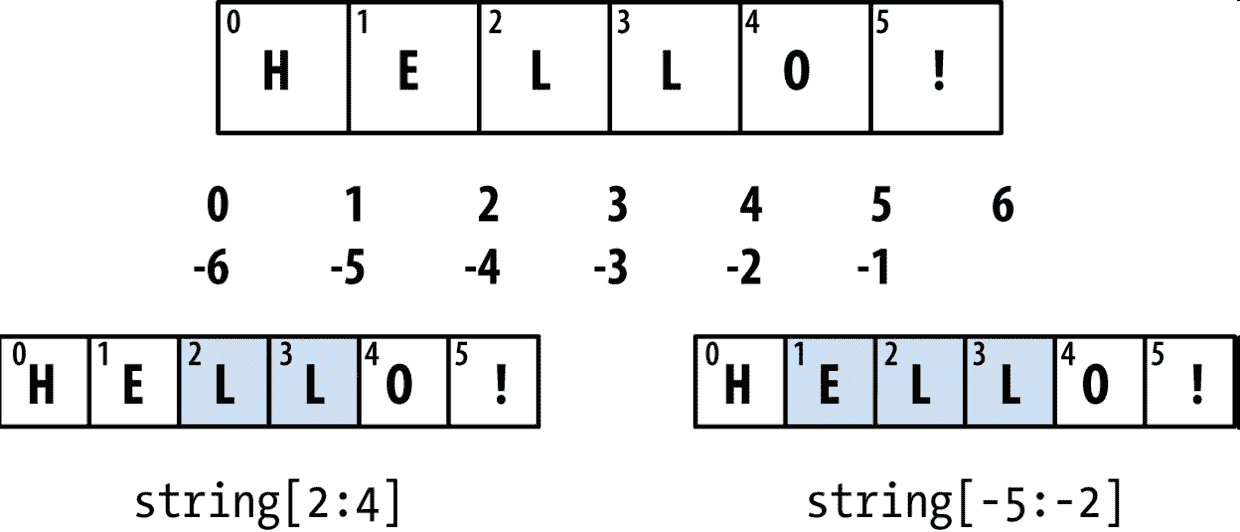In : seq[::2]
Out: [7, 3, 3, 6, 1]


In : seq[::-1]
Out: [1, 0, 6, 5, 3, 6, 3, 2, 7]


### 序列函数

Python 有一些有用的序列函数。

### enumerate函数

i = 0
for value in collection:
# do something with value
i += 1


for i, value in enumerate(collection):
# do something with value


In : some_list = ['foo', 'bar', 'baz']

In : mapping = {}

In : for i, v in enumerate(some_list):
....:     mapping[v] = i

In : mapping
Out: {'bar': 1, 'baz': 2, 'foo': 0}


### sorted函数

sorted函数可以从任意序列的元素返回一个新的排好序的列表：

In : sorted([7, 1, 2, 6, 0, 3, 2])
Out: [0, 1, 2, 2, 3, 6, 7]

In : sorted('horse race')
Out: [' ', 'a', 'c', 'e', 'e', 'h', 'o', 'r', 'r', 's']


sorted函数可以接受和sort相同的参数。

### zip函数

zip可以将多个列表、元组或其它序列成对组合成一个元组列表：

In : seq1 = ['foo', 'bar', 'baz']

In : seq2 = ['one', 'two', 'three']

In : zipped = zip(seq1, seq2)

In : list(zipped)
Out: [('foo', 'one'), ('bar', 'two'), ('baz', 'three')]


zip可以处理任意多的序列，元素的个数取决于最短的序列：

In : seq3 = [False, True]

In : list(zip(seq1, seq2, seq3))
Out: [('foo', 'one', False), ('bar', 'two', True)]


zip的常见用法之一是同时迭代多个序列，可能结合enumerate使用：

In : for i, (a, b) in enumerate(zip(seq1, seq2)):
....:     print('{0}: {1}, {2}'.format(i, a, b))
....:
0: foo, one
1: bar, two
2: baz, three


In : pitchers = [('Nolan', 'Ryan'), ('Roger', 'Clemens'),
....:             ('Schilling', 'Curt')]

In : first_names, last_names = zip(*pitchers)

In : first_names
Out: ('Nolan', 'Roger', 'Schilling')

In : last_names
Out: ('Ryan', 'Clemens', 'Curt')


### reversed函数

reversed可以从后向前迭代一个序列：

In : list(reversed(range(10)))
Out: [9, 8, 7, 6, 5, 4, 3, 2, 1, 0]


### 字典

In : empty_dict = {}

In : d1 = {'a' : 'some value', 'b' : [1, 2, 3, 4]}

In : d1
Out: {'a': 'some value', 'b': [1, 2, 3, 4]}


In : d1 = 'an integer'

In : d1
Out: {'a': 'some value', 'b': [1, 2, 3, 4], 7: 'an integer'}

In : d1['b']
Out: [1, 2, 3, 4]


In : 'b' in d1
Out: True


In : d1 = 'some value'

In : d1
Out:
{'a': 'some value',
'b': [1, 2, 3, 4],
7: 'an integer',
5: 'some value'}

In : d1['dummy'] = 'another value'

In : d1
Out:
{'a': 'some value',
'b': [1, 2, 3, 4],
7: 'an integer',
5: 'some value',
'dummy': 'another value'}

In : del d1

In : d1
Out:
{'a': 'some value',
'b': [1, 2, 3, 4],
7: 'an integer',
'dummy': 'another value'}

In : ret = d1.pop('dummy')

In : ret
Out: 'another value'

In : d1
Out: {'a': 'some value', 'b': [1, 2, 3, 4], 7: 'an integer'}


keysvalues是字典的键和值的迭代器方法。虽然键值对没有顺序，这两个方法可以用相同的顺序输出键和值：

In : list(d1.keys())
Out: ['a', 'b', 7]

In : list(d1.values())
Out: ['some value', [1, 2, 3, 4], 'an integer']


update方法可以将一个字典与另一个融合：

In : d1.update({'b' : 'foo', 'c' : 12})

In : d1
Out: {'a': 'some value', 'b': 'foo', 7: 'an integer', 'c': 12}


update方法是原地改变字典，因此任何传递给update的键的旧的值都会被舍弃。

### 用序列创建字典

mapping = {}
for key, value in zip(key_list, value_list):
mapping[key] = value


In : mapping = dict(zip(range(5), reversed(range(5))))

In : mapping
Out: {0: 4, 1: 3, 2: 2, 3: 1, 4: 0}


### 默认值

if key in some_dict:
value = some_dict[key]
else:
value = default_value


value = some_dict.get(key, default_value)


get默认会返回None，如果不存在键，pop会抛出一个例外。关于设定值，常见的情况是在字典的值是属于其它集合，如列表。例如，你可以通过首字母，将一个列表中的单词分类：

In : words = ['apple', 'bat', 'bar', 'atom', 'book']

In : by_letter = {}

In : for word in words:
.....:     letter = word
.....:     if letter not in by_letter:
.....:         by_letter[letter] = [word]
.....:     else:
.....:         by_letter[letter].append(word)
.....:

In : by_letter
Out: {'a': ['apple', 'atom'], 'b': ['bat', 'bar', 'book']}


setdefault方法就正是干这个的。前面的for循环可以改写为：

for word in words:
letter = word
by_letter.setdefault(letter, []).append(word)


collections模块有一个很有用的类，defaultdict，它可以进一步简化上面。传递类型或函数以生成每个位置的默认值：

from collections import defaultdict
by_letter = defaultdict(list)
for word in words:
by_letter[word].append(word)


### 有效的键类型

In : hash('string')
Out: 5023931463650008331

In : hash((1, 2, (2, 3)))
Out: 1097636502276347782

In : hash((1, 2, [2, 3])) # fails because lists are mutable
---------------------------------------------------------------------------
TypeError                                 Traceback (most recent call last)
<ipython-input-129-800cd14ba8be> in <module>()
----> 1 hash((1, 2, [2, 3])) # fails because lists are mutable
TypeError: unhashable type: 'list'


In : d = {}

In : d[tuple([1, 2, 3])] = 5

In : d
Out: {(1, 2, 3): 5}


### 集合

In : set([2, 2, 2, 1, 3, 3])
Out: {1, 2, 3}

In : {2, 2, 2, 1, 3, 3}
Out: {1, 2, 3}


In : a = {1, 2, 3, 4, 5}

In : b = {3, 4, 5, 6, 7, 8}


In : a.union(b)
Out: {1, 2, 3, 4, 5, 6, 7, 8}

In : a | b
Out: {1, 2, 3, 4, 5, 6, 7, 8}


In : a.intersection(b)
Out: {3, 4, 5}

In : a & b
Out: {3, 4, 5}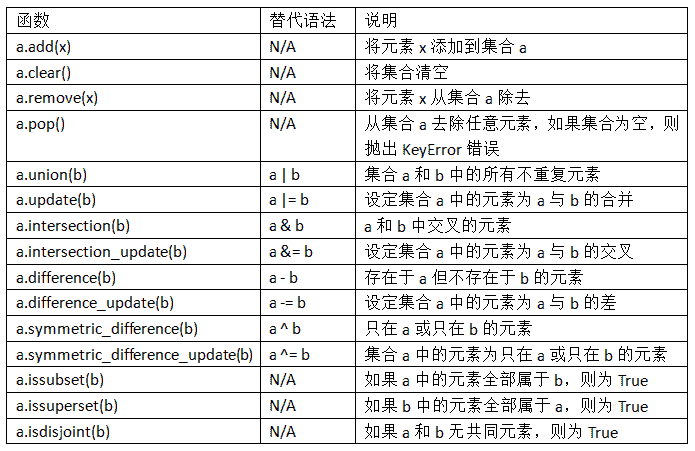In : c = a.copy()

In : c |= b

In : c
Out: {1, 2, 3, 4, 5, 6, 7, 8}

In : d = a.copy()

In : d &= b

In : d
Out: {3, 4, 5}


In : my_data = [1, 2, 3, 4]

In : my_set = {tuple(my_data)}

In : my_set
Out: {(1, 2, 3, 4)}


In : a_set = {1, 2, 3, 4, 5}

In : {1, 2, 3}.issubset(a_set)
Out: True

In : a_set.issuperset({1, 2, 3})
Out: True


In : {1, 2, 3} == {3, 2, 1}
Out: True


### 列表、集合和字典推导式

[expr for val in collection if condition]


result = []
for val in collection:
if condition:
result.append(expr)


In : strings = ['a', 'as', 'bat', 'car', 'dove', 'python']

In : [x.upper() for x in strings if len(x) > 2]
Out: ['BAT', 'CAR', 'DOVE', 'PYTHON']


dict_comp = {key-expr : value-expr for value in collection if condition}


set_comp = {expr for value in collection if condition}


In : unique_lengths = {len(x) for x in strings}

In : unique_lengths
Out: {1, 2, 3, 4, 6}


map函数可以进一步简化：

In : set(map(len, strings))
Out: {1, 2, 3, 4, 6}


In : loc_mapping = {val : index for index, val in enumerate(strings)}

In : loc_mapping
Out: {'a': 0, 'as': 1, 'bat': 2, 'car': 3, 'dove': 4, 'python': 5}


### 嵌套列表推导式

In : all_data = [['John', 'Emily', 'Michael', 'Mary', 'Steven'],
.....:             ['Maria', 'Juan', 'Javier', 'Natalia', 'Pilar']]


names_of_interest = []
for names in all_data:
enough_es = [name for name in names if name.count('e') >= 2]
names_of_interest.extend(enough_es)


In : result = [name for names in all_data for name in names
.....:           if name.count('e') >= 2]

In : result
Out: ['Steven']


In : some_tuples = [(1, 2, 3), (4, 5, 6), (7, 8, 9)]

In : flattened = [x for tup in some_tuples for x in tup]

In : flattened
Out: [1, 2, 3, 4, 5, 6, 7, 8, 9]


flattened = []

for tup in some_tuples:
for x in tup:
flattened.append(x)


In : [[x for x in tup] for tup in some_tuples]
Out: [[1, 2, 3], [4, 5, 6], [7, 8, 9]]


## 3.2 函数

def my_function(x, y, z=1.5):
if z > 1:
return z * (x + y)
else:
return z / (x + y)


my_function(5, 6, z=0.7)
my_function(3.14, 7, 3.5)
my_function(10, 20)


python my_function(x=5, y=6, z=7) my_function(y=6, x=5, z=7)

### 命名空间、作用域，和局部函数

def func():
a = []
for i in range(5):
a.append(i)


a = []
def func():
for i in range(5):
a.append(i)


In : a = None

In : def bind_a_variable():
.....:     global a
.....:     a = []
.....: bind_a_variable()
.....:

In : print(a)
[]


### 返回多个值

def f():
a = 5
b = 6
c = 7
return a, b, c

a, b, c = f()


return_value = f()


def f():
a = 5
b = 6
c = 7
return {'a' : a, 'b' : b, 'c' : c}


### 函数也是对象

In : states = ['   Alabama ', 'Georgia!', 'Georgia', 'georgia', 'FlOrIda',
.....:           'south   carolina##', 'West virginia?']


import re

def clean_strings(strings):
result = []
for value in strings:
value = value.strip()
value = re.sub('[!#?]', '', value)
value = value.title()
result.append(value)
return result


In : clean_strings(states)
Out:
['Alabama',
'Georgia',
'Georgia',
'Georgia',
'Florida',
'South   Carolina',
'West Virginia']


def remove_punctuation(value):
return re.sub('[!#?]', '', value)

clean_ops = [str.strip, remove_punctuation, str.title]

def clean_strings(strings, ops):
result = []
for value in strings:
for function in ops:
value = function(value)
result.append(value)
return result


In : clean_strings(states, clean_ops)
Out:
['Alabama',
'Georgia',
'Georgia',
'Georgia',
'Florida',
'South   Carolina',
'West Virginia']


In : for x in map(remove_punctuation, states):
.....:     print(x)
Alabama
Georgia
Georgia
georgia
FlOrIda
south   carolina
West virginia


### 匿名（lambda）函数

Python 支持一种被称为匿名的、或 lambda 函数。它仅由单条语句组成，该语句的结果就是返回值。它是通过lambda关键字定义的，这个关键字没有别的含义，仅仅是说“我们正在声明的是一个匿名函数”。

def short_function(x):
return x * 2

equiv_anon = lambda x: x * 2


def apply_to_list(some_list, f):
return [f(x) for x in some_list]

ints = [4, 0, 1, 5, 6]
apply_to_list(ints, lambda x: x * 2)


In : strings = ['foo', 'card', 'bar', 'aaaa', 'abab']


In : strings.sort(key=lambda x: len(set(list(x))))

In : strings
Out: ['aaaa', 'foo', 'abab', 'bar', 'card']


### 柯里化：部分参数应用

def add_numbers(x, y):
return x + y


add_five = lambda y: add_numbers(5, y)


add_numbers的第二个参数称为“柯里化的”（curried）。这里没什么特别花哨的东西，因为我们其实就只是定义了一个可以调用现有函数的新函数而已。内置的functools模块可以用partial函数将此过程简化：

from functools import partial


### 生成器

In : some_dict = {'a': 1, 'b': 2, 'c': 3}

In : for key in some_dict:
.....:     print(key)
a
b
c


In : dict_iterator = iter(some_dict)

In : dict_iterator
Out: <dict_keyiterator at 0x7fbbd5a9f908>


In : list(dict_iterator)
Out: ['a', 'b', 'c']


def squares(n=10):
print('Generating squares from 1 to {0}'.format(n ** 2))
for i in range(1, n + 1):
yield i ** 2


In : gen = squares()

In : gen
Out: <generator object squares at 0x7fbbd5ab4570>


In : for x in gen:
.....:     print(x, end=' ')
Generating squares from 1 to 100
1 4 9 16 25 36 49 64 81 100


### 生成器表达式

In : gen = (x ** 2 for x in range(100))

In : gen
Out: <generator object <genexpr> at 0x7fbbd5ab29e8>


def _make_gen():
for x in range(100):
yield x ** 2
gen = _make_gen()


In : sum(x ** 2 for x in range(100))
Out: 328350

In : dict((i, i **2) for i in range(5))
Out: {0: 0, 1: 1, 2: 4, 3: 9, 4: 16}


### itertools模块

In : import itertools

In : first_letter = lambda x: x

In : names = ['Alan', 'Adam', 'Wes', 'Will', 'Albert', 'Steven']

In : for letter, names in itertools.groupby(names, first_letter):
.....:     print(letter, list(names)) # names is a generator
W ['Wes', 'Will']
A ['Albert']
S ['Steven']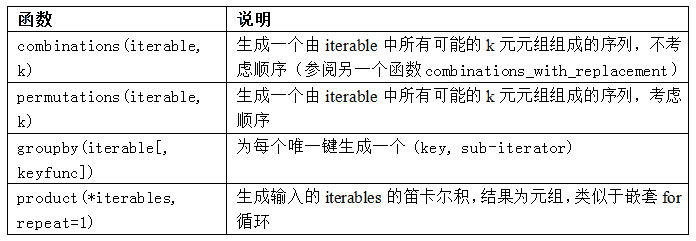### 错误和异常处理

In : float('1.2345')
Out: 1.2345

In : float('something')
---------------------------------------------------------------------------
ValueError                                Traceback (most recent call last)
<ipython-input-198-439904410854> in <module>()
----> 1 float('something')
ValueError: could not convert string to float: 'something'


def attempt_float(x):
try:
return float(x)
except:
return x


float(x)抛出异常时，才会执行except的部分：

In : attempt_float('1.2345')
Out: 1.2345

In : attempt_float('something')
Out: 'something'


In : float((1, 2))
---------------------------------------------------------------------------
TypeError                                 Traceback (most recent call last)
<ipython-input-202-842079ebb635> in <module>()
----> 1 float((1, 2))
TypeError: float() argument must be a string or a number, not 'tuple'


def attempt_float(x):
try:
return float(x)
except ValueError:
return x


In : attempt_float((1, 2))
---------------------------------------------------------------------------
TypeError                                 Traceback (most recent call last)
----> 1 attempt_float((1, 2))
<ipython-input-203-3e06b8379b6b> in attempt_float(x)
1 def attempt_float(x):
2     try:
----> 3         return float(x)
4     except ValueError:
5         return x
TypeError: float() argument must be a string or a number, not 'tuple'


def attempt_float(x):
try:
return float(x)
except (TypeError, ValueError):
return x


f = open(path, 'w')

try:
write_to_file(f)
finally:
f.close()


f = open(path, 'w')

try:
write_to_file(f)
except:
print('Failed')
else:
print('Succeeded')
finally:
f.close()


### IPython 的异常

In : %run examples/ipython_bug.py
---------------------------------------------------------------------------
AssertionError                            Traceback (most recent call last)
/home/wesm/code/pydata-book/examples/ipython_bug.py in <module>()
13     throws_an_exception()
14
---> 15 calling_things()

/home/wesm/code/pydata-book/examples/ipython_bug.py in calling_things()
11 def calling_things():
12     works_fine()
---> 13     throws_an_exception()
14
15 calling_things()

/home/wesm/code/pydata-book/examples/ipython_bug.py in throws_an_exception()
7     a = 5
8     b = 6
----> 9     assert(a + b == 10)
10
11 def calling_things():

AssertionError:


## 3.3 文件和操作系统

In : path = 'examples/segismundo.txt'

In : f = open(path)


for line in f:
pass


In : lines = [x.rstrip() for x in open(path)]

In : lines
Out:
['Sueña el rico en su riqueza,',
'',
'su miseria y su pobreza;',
'',
'sueña el que a medrar empieza,',
'sueña el que afana y pretende,',
'sueña el que agravia y ofende,',
'',
'y en el mundo, en conclusión,',
'todos sueñan lo que son,',
'aunque ninguno lo entiende.',
'']


In : f.close()


with语句可以可以更容易地清理打开的文件：

In : with open(path) as f:
.....:     lines = [x.rstrip() for x in f]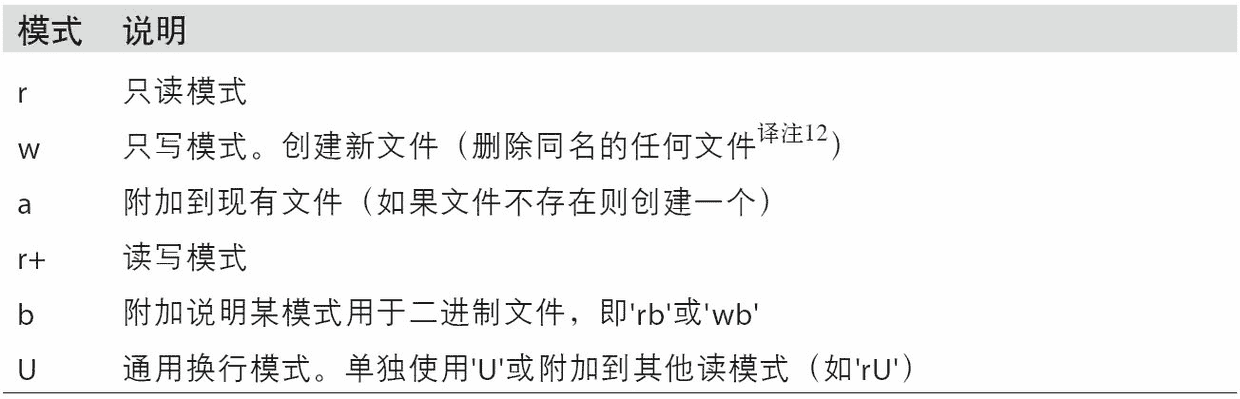In : f = open(path)

Out: 'Sueña el r'

In : f2 = open(path, 'rb')  # Binary mode

Out: b'Sue\xc3\xb1a el '


read模式会将文件句柄的位置提前，提前的数量是读取的字节数。tell可以给出当前的位置：

In : f.tell()
Out: 11

In : f2.tell()
Out: 10


In : import sys

In : sys.getdefaultencoding()
Out: 'utf-8'


seek将文件位置更改为文件中的指定字节：

In : f.seek(3)
Out: 3

Out: 'ñ'


In : f.close()

In : f2.close()


In : with open('tmp.txt', 'w') as handle:
.....:     handle.writelines(x for x in open(path) if len(x) > 1)

In : with open('tmp.txt') as f:

In : lines
Out:
['Sueña el rico en su riqueza,\n',
'su miseria y su pobreza;\n',
'sueña el que a medrar empieza,\n',
'sueña el que afana y pretende,\n',
'sueña el que agravia y ofende,\n',
'y en el mundo, en conclusión,\n',
'todos sueñan lo que son,\n',
'aunque ninguno lo entiende.\n']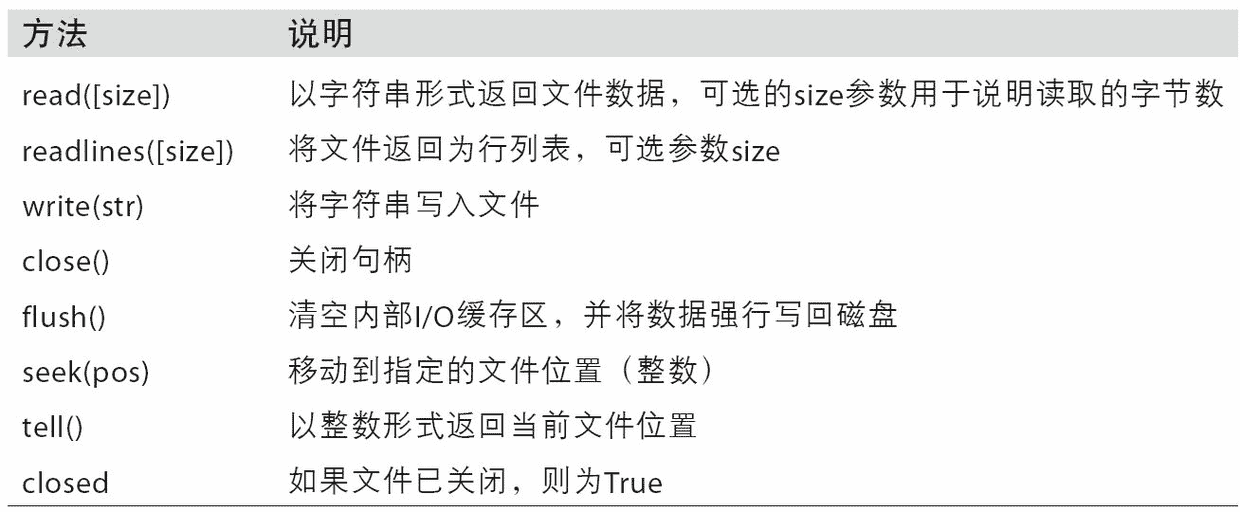### 文件的字节和 Unicode

Python 文件的默认操作是“文本模式”，也就是说，你需要处理 Python 的字符串（即 Unicode）。它与“二进制模式”相对，文件模式加一个b。我们来看上一节的文件（UTF-8 编码、包含非 ASCII 字符）：

In : with open(path) as f:

In : chars
Out: 'Sueña el r'


UTF-8 是长度可变的 Unicode 编码，所以当我从文件请求一定数量的字符时，Python 会从文件读取足够多（可能少至 10 或多至 40 字节）的字节进行解码。如果以rb模式打开文件，则读取确切的请求字节数：

In : with open(path, 'rb') as f:

In : data
Out: b'Sue\xc3\xb1a el '


In : data.decode('utf8')
Out: 'Sueña el '

In : data[:4].decode('utf8')
---------------------------------------------------------------------------
UnicodeDecodeError                        Traceback (most recent call last)
<ipython-input-235-300e0af10bb7> in <module>()
----> 1 data[:4].decode('utf8')
UnicodeDecodeError: 'utf-8' codec can't decode byte 0xc3 in position 3: unexpecte
d end of data


In : sink_path = 'sink.txt'

In : with open(path) as source:
.....:     with open(sink_path, 'xt', encoding='iso-8859-1') as sink:

In : with open(sink_path, encoding='iso-8859-1') as f:
Sueña el r


In : f = open(path)

Out: 'Sueña'

In : f.seek(4)
Out: 4

---------------------------------------------------------------------------
UnicodeDecodeError                        Traceback (most recent call last)
<ipython-input-243-7841103e33f5> in <module>()
/miniconda/envs/book-env/lib/python3.6/codecs.py in decode(self, input, final)
319         # decode input (taking the buffer into account)
320         data = self.buffer + input
--> 321         (result, consumed) = self._buffer_decode(data, self.errors, final
)
322         # keep undecoded input until the next call
323         self.buffer = data[consumed:]
UnicodeDecodeError: 'utf-8' codec can't decode byte 0xb1 in position 0: invalid s
tart byte

In : f.close()